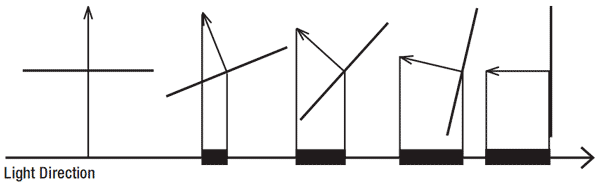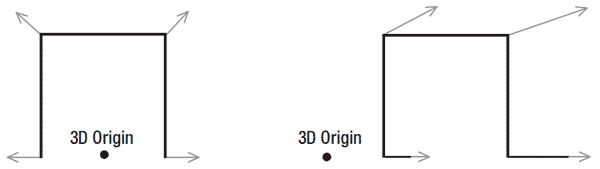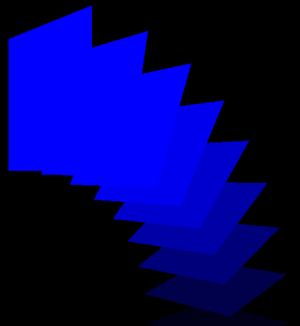## 工作原理

float4x4 xWorld;
float4x4 xView;
float4x4 xProjection;
float xAmbient; float3 xLightDirection;

struct VSVertexToPixel
{
float4 Position : POSITION;
float LightingFactor : TEXCOORD0;
};

struct VSPixelToFrame
{
float4 Color : COLOR0;
};VSVertexToPixel VSVertexShader(float4 inPos: POSITION0, float3 inNormal: NORMAL0)
{
VSVertexToPixel Output = (VSVertexToPixel)0;

float4x4 preViewProjection = mul(xView, xProjection);
float4x4 preWorldViewProjection = mul(xWorld, preViewProjection);

Output.Position = mul(inPos, preWorldViewProjection);
float3 normal = normalize(inNormal);
Output.LightFactor = dot(rotNormal, -xLightDirection);

return Output;
}

### 使用世界矩阵时确保正确的光照float3 normal = normalize(inNormal);
float3x3 rotMatrix = (float3x3)xWorld;
float3 rotNormal = mul(normal, rotMatrix);
Output.LightFactor = dot(rotNormal, -xLightDirection);

VSPixelToFrame VSPixelShader(VSVertexToPixel PSIn) : COLOR0
{
VSPixelToFrame Output = (VSPixelToFrame)0;

float4 baseColor = float4(0,0,1,1);
Output.Color = baseColor*(PSIn.LightFactor+xAmbient);

return Output;
}

### 定义technique

technique VertexShading
{
pass Pass0
{
}
}

### XNA代码

effect = content.Load<Effect>("vertexshading");

effect.CurrentTechnique = effect.Techniques["VertexShading"];
effect.Parameters["xWorld"].SetValue(Matrix.Identity);
effect.Parameters["xView"].SetValue(fpsCam.ViewMatrix);
effect.Parameters["xProjection"].SetValue(fpsCam.ProjectionMatrix);
effect.Parameters["xLightDirection"].SetValue(new Vector3(1, 0, 0));

effect.Begin();
foreach (EffectPass pass in effect.CurrentTechnique.Passes)
{
pass.Begin();
device.VertexDeclaration = myVertexDeclaration;
device.DrawUserPrimitives<VertexPositionNormalTexture>(PrimitiveType.TriangleList, vertices, 0, 2);
pass.End();
}
effect.End();

## 代码

XNA代码绘制对象的多个实例。因为使用了不同的世界矩阵，这些对象会绘制在不同位置。

effect.CurrentTechnique = effect.Techniques["VertexShading"];

effect.Parameters["xView"].SetValue(fpsCam.ViewMatrix);
effect.Parameters["xProjection"].SetValue(fpsCam.ProjectionMatrix);
effect.Parameters["xLightDirection"].SetValue(new Vector3(1, 0, 0));
effect.Parameters["xAmbient"].SetValue(0.0f);

for (int i = 0; i < 9; i++)
{
Matrix world = Matrix.CreateTranslation(4, 0, 0) * Matrix.CreateRotationZ((float)i * MathHelper.PiOver2 / 8.0f);

effect.Parameters["xWorld"].SetValue(world);

effect.Begin();
foreach (EffectPass pass in effect.CurrentTechnique.Passes)
{
pass.Begin();
device.VertexDeclaration = myVertexDeclaration;
device.DrawUserPrimitives<VertexPositionNormalTexture> (PrimitiveType.TriangleList, vertices, 0, 2);
pass.End();
}
effect.End();
}

float4x4 xWorld;
float4x4 xView;
float4x4 xProjection;
float xAmbient;
float3 xLightDirection;

struct VSVertexToPixel
{
float4 Position : POSITION;
float LightFactor : TEXCOORD0;
};

struct VSPixelToFrame
{
float4 Color : COLOR0;
}

VSVertexToPixel VSVertexShader(float4 inPos: POSITION0, float3 inNormal: NORMAL0)
{
VSVertexToPixel Output = (VSVertexToPixel)0;

float4x4 preViewProjection = mul(xView, xProjection);
float4x4 preWorldViewProjection = mul(xWorld, preViewProjection);
Output.Position = mul(inPos, preWorldViewProjection);
float3 normal = normalize(inNormal);
float3x3 rotMatrix = (float3x3)xWorld;
float3 rotNormal = mul(normal, rotMatrix);
Output.LightFactor = dot(rotNormal, -xLightDirection);

return Output;
}

{
VSPixelToFrame Output = (VSPixelToFrame)0;

float4 baseColor = float4(0,0,1,1);
Output.Color = baseColor*(PSIn.LightFactor+xAmbient);

return Output;
}

{
pass Pass0
{
}